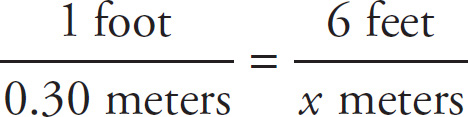# SAT Math Multiple Choice Question 167: Answer and Explanation

### Test Information

Question: 167

2. Taylor is 6 feet tall. If 1 foot is equal to approximately 0.3 meters, then which of the following is closest to Taylor's height in meters?

• A. 1.8
• B. 2
• C. 18
• D. 20

A Set up a proportion:. Cross-multiply to get x = 6 × 0.30 = 1.8 meters. Therefore, the correct answer is (A).